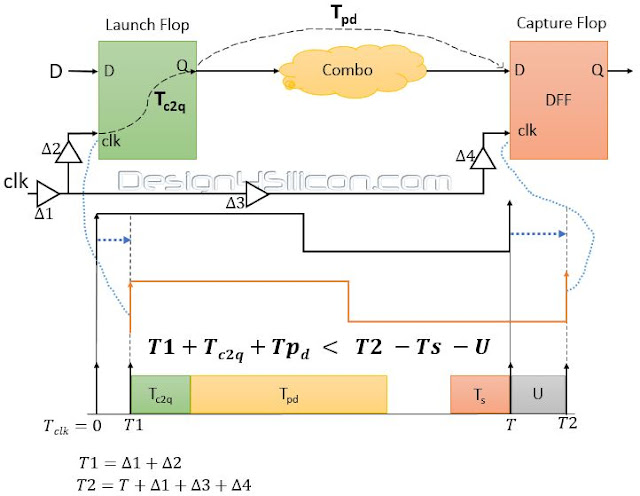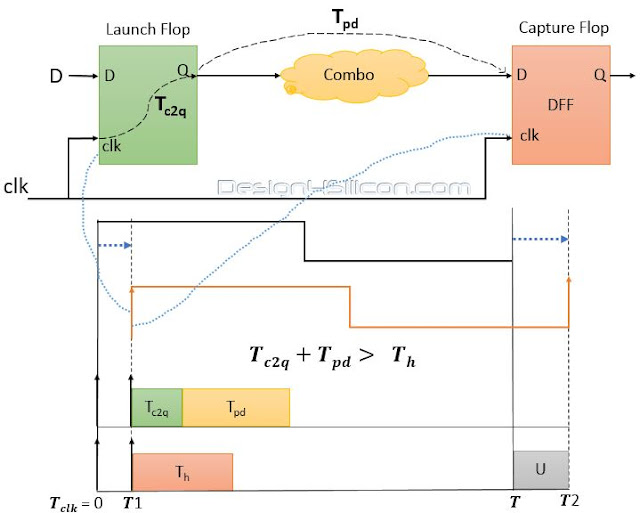## Wednesday, March 9, 2016

### Basics of Setup and Hold Part -2

Clock plays very crucial role in sequential circuits but Skew and Jitter in clocks can make the stable circuit to unstable. Let’s discuss this one by one what are skew and jitter also how they affect the sequential circuit.

CLOCK SKEW
In circuit designs, clock skew is a phenomenon in synchronous circuits in which the same sourced clock signal arrives at different components (generally latches or flip-flops) at different times. This is due to interconnects delay and clock buffer delay.Clock Skew
Suppose when there is no clock buffers then clock edge reaches at launch flop at time t=0 and due to clock buffer 1 and 2 in the path of launch flop it reaches at time t = D1 + D2.
Similar for capture flop when there is no clock buffers then clock edge reaches at time t = T but due to clock buffers delay in clock path it reaches at time
t = T + D1 + D3 + D4.
If we assume clock edge reaches in launch flop at time t = T1 and next edge reaches at capture flop at time t = T2 then we can say
CLOCK SKEW = |T2 - T1 - T|
Or
CLOCK SKEW = |(D1+D3+D4) - (D1+D2)|

CLOCK JITTER
Deviation or displacement of a periodic signal from its true position is called jitter. Electromagnetic interference and crosstalk are major cause for jitter in clock.Clock Jitter
While calculation of setup delay we subtract jitter time or uncertainty (U) from clock period (T). So actual time period is now T - U.

THE EFFECT OF 'SKEW' AND 'JITTER' IN SETUP & HOLD TIME ANALYSIS
Now let us look the effect of ‘clock skew’ and ‘clock jitter’ in setup and hold time analysis of two flop network. Setup delay equation from previous blog
Tc2q + Tpd  <  T - Ts
When there is jitter in clock then the setup time equation become
Tc2q + Tpd  <  T - Ts - U
Also consider the clock delay in network then it become
Tc2q + Tpd + (D1 + D2) <  (T + D1 + D3 + D4) - Ts - U
This can be understand by following timing diagram.Effect of Jitter and Skew in Setup Analysis

Hold delay is not much affected by clock jitter and skew because we analyze it at same clock edge. We can understand it by following timing diagram.Effect of Jitter and Skew on Hold Analysis

In NEXT blog there are some exercise questions asked in interviews and competitive exams. This also help in to better understanding of the concept of setup and hold time. Hope you enjoy them also.